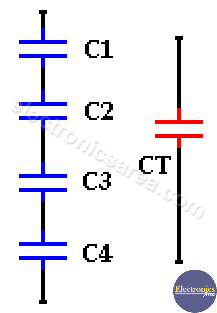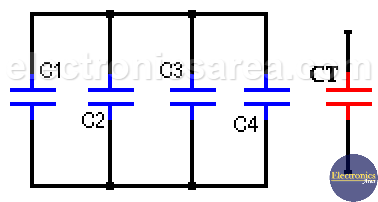# Capacitors in Series – Capacitors in Parallel

The capacitor is a device made up of two metal plates separated by an insulator called a dielectric. A dielectric or insulator is a material that prevents the passage of direct current between the plates, but allows the passage of alternating current signals to a degree that depends on their frequency.

There are two typical connection types between capacitors, and they are capacitors in series and capacitors parallel.

## Capacitors in series

Capacitors in series are capacitors connected one after the other. These capacitors can be replaced by a single equivalent capacitor that has a value that is the equivalent of those that are connected in series.

To obtain the value (capacitance) of this single equivalent capacitor, the following formula is used (for 4 capacitors):

1/Ceq = 1/C1+1/C2+1/C3+1/C4     or   Ceq = (C1 x C2 x C3 x C4) / (C1 + C2 + C3 + C4)

It is easy to make this calculation for any number of capacitors using the most general formula:

1/Ceq = 1/C1+1/C2+………+1/CN    or    Ceq = (C1 x C2 x …. x CN) / (C1 + C2 + ….. + CN)

Where N is the number of capacitors that are connected in series. See the diagram.For example:

If I have 2 capacitors connected in series, C1 = 470uF, C2 = 100uF. What is the capacitance of the equivalent capacitor?

Using the formula: Ceq = (C1 x C2) / (C1 + C2).

Ceq = (470uF x 100uF) / (470uF + 100uF) = 47000/570 = 82.456uF

## Capacitors in parallel

Capacitors in parallel are capacitors connected one beside the other. The terminals on either side of the capacitors are connected together (connected to the same point).

These capacitors can be replaced with a single equivalent capacitor with a value (capacitance) equivalent of those that are connected in parallel.

The following picture shows how to connect 4 capacitors in parallel. To find the equivalent capacitor value (CT)  we use the formula : CT = C1 + C2 + C3 + C4.

It is easy to make a calculation for any number of capacitors with the most general formula:

CT = C1 + C2 + ………+ CN, where N is the number of capacitors.

As we already notice, to get the equivalent capacitor value (capacitance) of a set of capacitors in parallel we only have to add the capacitors values (capacitance) of the original circuit.For example:

If I have 3 capacitors connected in parallel, C1 = 470uF, C2 = 1000uF and C3 = 100uF. What is the capacitance of the equivalent capacitor?

Using the formula: Ceq = C1 + C2 + C3.

Ceq = 470uF + 1000uF + 100uF = 1570uF.

capacitors-series-and-parallel

•
•
• 5
•
•
•
•
•
5
Shares
•
5
Shares
•
•
• 5
•
•
•
•